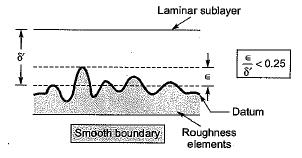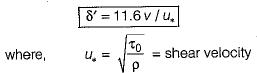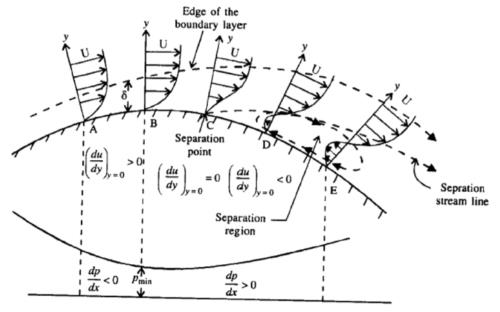Courses

# Test: Boundary Layer Theory - 1

## 10 Questions MCQ Test Fluid Mechanics | Test: Boundary Layer Theory - 1

Description
This mock test of Test: Boundary Layer Theory - 1 for Mechanical Engineering helps you for every Mechanical Engineering entrance exam. This contains 10 Multiple Choice Questions for Mechanical Engineering Test: Boundary Layer Theory - 1 (mcq) to study with solutions a complete question bank. The solved questions answers in this Test: Boundary Layer Theory - 1 quiz give you a good mix of easy questions and tough questions. Mechanical Engineering students definitely take this Test: Boundary Layer Theory - 1 exercise for a better result in the exam. You can find other Test: Boundary Layer Theory - 1 extra questions, long questions & short questions for Mechanical Engineering on EduRev as well by searching above.
QUESTION: 1

Solution:
QUESTION: 2

Solution:
QUESTION: 3

### Select the wrong statement pertaining to flow beyond the outer edge of boundary layer:

Solution:
QUESTION: 4

In a boundary layer developed along the flow, the pressure decreases along the downstream direction. The boundary layer thickness would

Solution:
QUESTION: 5

The laminar sublayer is

Solution:
QUESTION: 6

In a hydrodynamically smooth surface the roughness magnitude ∈ and laminar sublayer thickness δ' are related as

Solution:QUESTION: 7

The thickness of laminar sublayer δ' is given by

Solution:

The laminar sublayer is usually very thin and it! thickness δ' is found hv exneriments to beQUESTION: 8

The separation of boundary layer takes place when the pressure gradient is

Solution:

In advance pressure gradient boundary layer flow the boundary layer may at some section leave the boundary. This is called as separation and downstream of the separation section turbulent eddies exist and this disturbed region is called as wake.

QUESTION: 9

The boundary layer separates from the surface when

Solution:

dp/dx ≠ 0QUESTION: 10

Some times and under conditions, the boundary layer will leave the surface and curl up into a vortex or whirl pool. This phenomenon is known as

Solution: